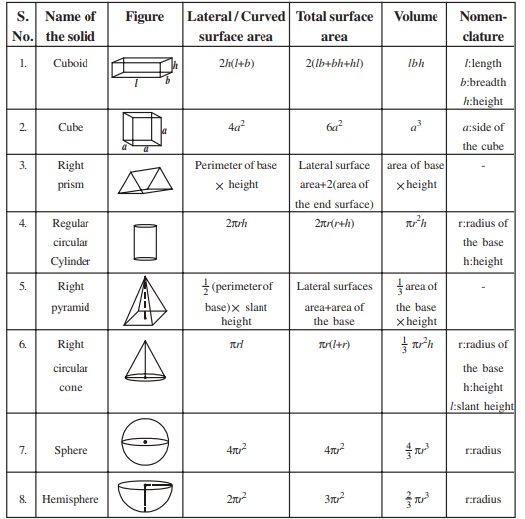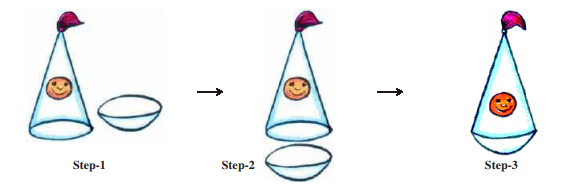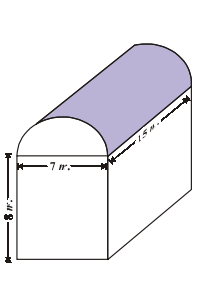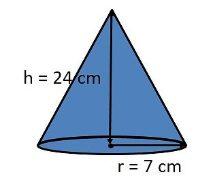# AP SSC Class 10 Maths Chapter 10 Mensuration

AP SSC Class 10 Maths Chapter 10 Mensuration is an interesting chapter. Mensuration is a branch of geometry that deals with the measurement of length, volume and area. To master mensuration, one needs to remember the surface area and volumes of different solid shapes. For your reference, we have provided formulas of different solid shapes.

Here, in this AP SSC 10th Class Maths Chapter 10 Mensuration notes we have given the key highlights and the excercise examples for the students to practise and also understand the concepts that are taught in class under the subject.## Surface Area and Volume Of Combination Of Solids

While calculating the surface area of a combination of solids, the surface area of the two constituents cannot be added, because some part of the surface area, disappears while joining them. To better understand, let us look at the example below.

Example: Deepa makes a toy putting together a hemisphere and a cone. So how do we find the total surface area of the newly formed toy?The total surface area of the newly formed toy is given by

TSA of the toy = CSA of Hemisphere + CSA of the cone

TSA and CSA stand for total surface and curved surface area respectively.

The volume of the solid formed by joining two basic solids is the sum of the volumes of the constituents. Let us look at the example below, to better understandSuresh runs an industry in a shed which is in the shape of a cuboid surmounted by a half cylinder as shown in the figure. The volume of air inside the shed (when there are no people or machinery) is given by the volume of air inside the cuboid and inside the half cylinder taken together as follows

Required Volume = Volume of the cuboid + ½ volume of the cylinder

In the next section, let us look at a few solutions to the chapter questions to better understand.

## Class 10 Maths Chapter 10 Mensuration Solutions

1. A joker’s cap is in the form of a right circular cone whose base radius is 7cm and height is 24 cm. Find the area of the sheet required to make 15 such caps.Solution:

The curved surface will determine the sheet required.

The curved surface area is given by the formula $\pi rl$.

According to Pythagoras theorem,

$l^{2}=h^{2}+r^{2}$

Substituting the values, we get

$l^{2}=24^{2}+7^{2}$ $l^{2}=625$ $l=\sqrt{625}=25\, cm$

Now substituting the value of l in the formula, we can determine the curved surface area as follows:

=$\frac{22}{7}\times 7\times 25$

= 550 $cm^2$

The curved surface of 15 such caps is $15\times 550=8250cm^2$

Stay tuned to BYJU’S to get the latest notification on SSC exams along with AP SSC model papers, exam pattern, marking scheme and more.